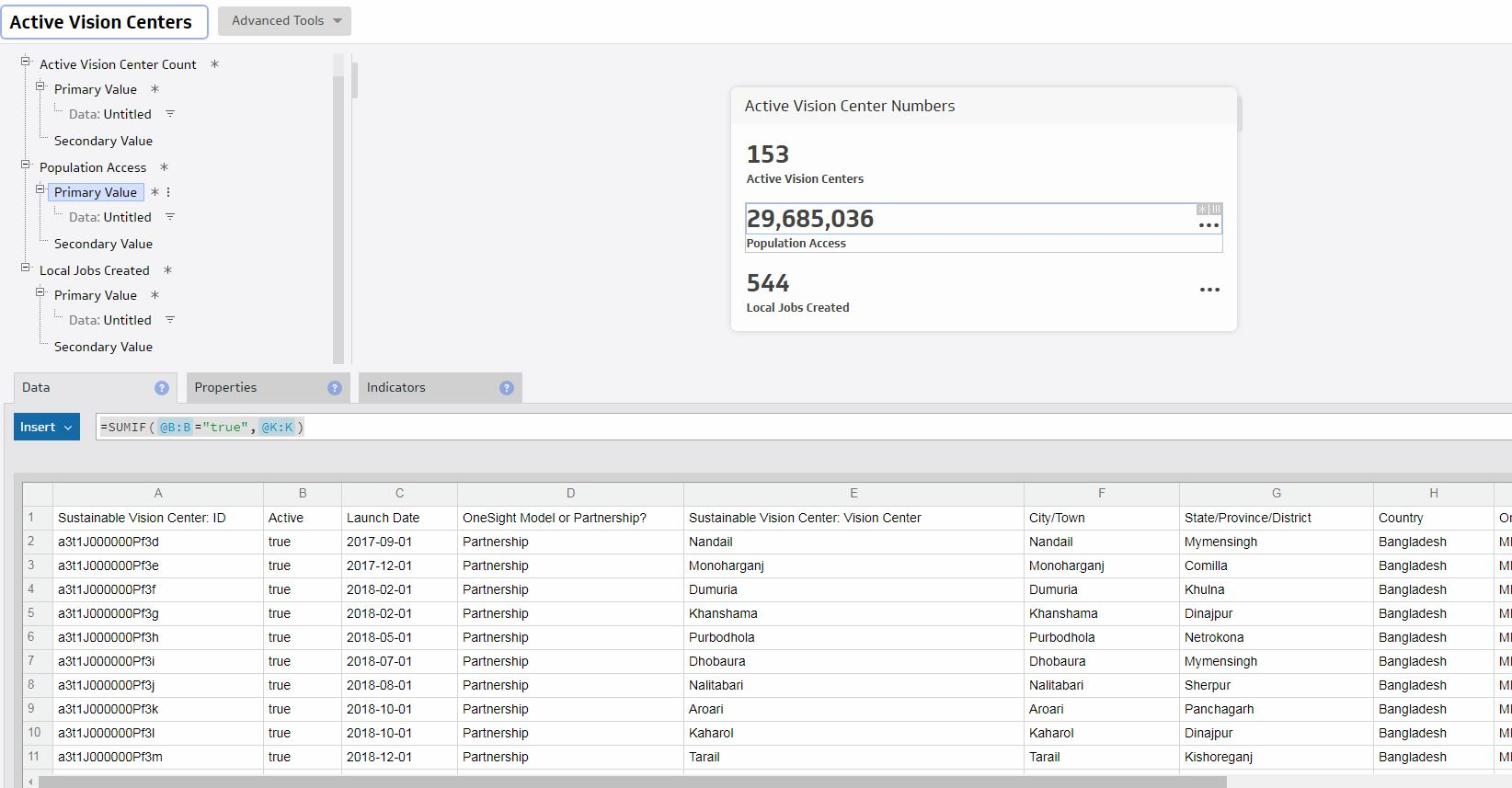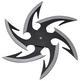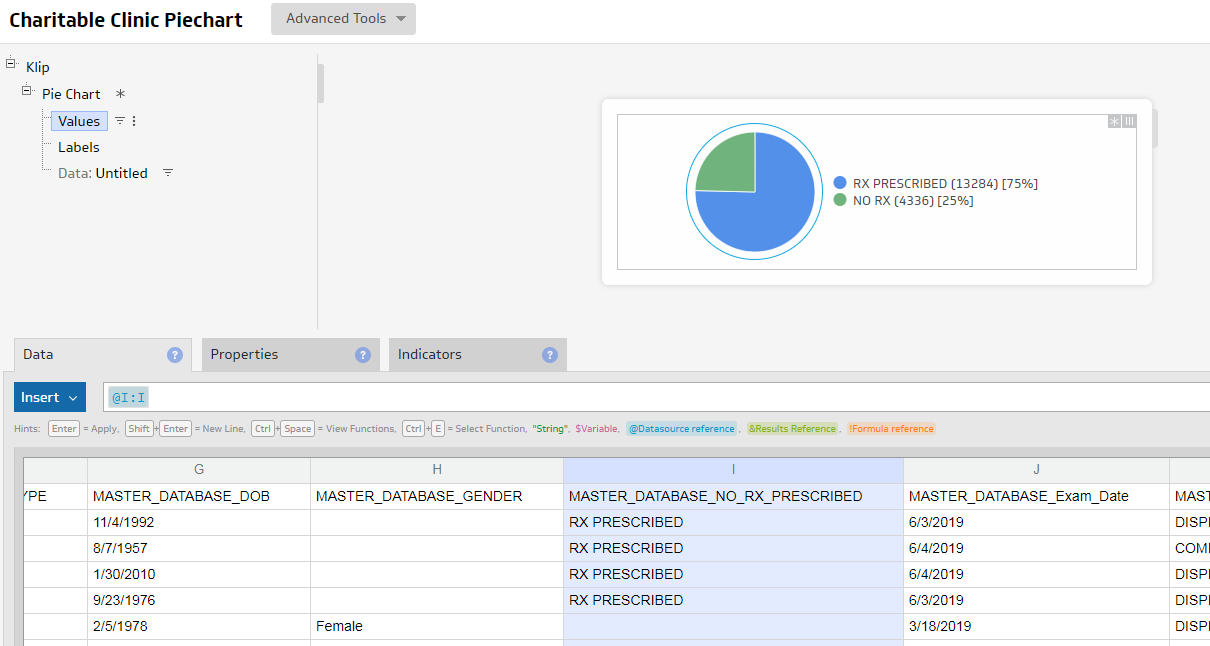Hi ,

I am working on a user input controlled klip with value pairs as my component. For some reason only the top value in my screenshot is working when using the user input control.

The user input control variable is column H , the list of countries, called "sustainablecountries"

Each number also needs to be calculated from only active vision centers. This is determined by "true" in column B.

For the top number "Active Vision Centers" - =IF(@B:B="true",1,0)

This contains hidden data @H:H (Country) where the filter is matching "sustainablecountries", and does not match "Country". This is being aggregated by Sum

For "Population Access" =SUMIF(@B:B="true",@K:K)

I set up the hidden data the same exact was as above. Even if I aggregate or turn off aggregation, it simply comes up as 0 when the user input control is used.

For "Local Jobs Created" =SUMIF(@B:B="true"@L:L)

everything the same as above

I am not sure why the top number is working when using the input control but the other values or not. My formulas are not showing any errors.Thanks!

•Janice Janczyn

Hi Kevin,

Hidden data and its associated subcomponents must be aligned to work correctly. Evaluate each formula to see how many values they return and if the subcomponents do not return the same number of items, the data is not aligned.

In the formula for Active Vision Centers, the IF returns 1 value for each item in column B. Assuming all the columns in your data have the same number of rows, this means it returns 1 value for each value in column H. In this case, your data is aligned 1 to 1 and the results are correct

However, in the formula for Population Access, the SUMIF will always return only 1 value, regardless of the number if items in columns B or K. In this case, the data is not aligned with column H. Instead of using SUMIF, use the IF construct again as you did for Active Vision Centers: IF( @B:B = "true", @K:K, 0 ), Set Aggregation > Sum. Similarly for Local Jobs Created.

Note that you do not need to prefix the equals sign (=) onto a formula, that is, use IF(@B:B="true",1,0) instead of =IF(@B:B="true",1,0)

Thank you,
Janice

•Kevin Burke

Hey Janice,

I was unaware I could use the entire column (@K:K) in the IF condition formula.

Much thanks for you help,

Kevin

•Kevin Burke

Hey Janice,

Trying this with a pie chart now and having similar trouble. I guess I am confused on when to use hidden data to set the variable. For the pie chart should I just be adding the variable on the filter on the values?

Thanks,

Kevin

•Kevin Burke

Sorry - see attached image.

Values = @I:I , for values I am filtering out the header and some other values to only leave RX PRESCRIBED and NO RX

Labels = @I:I , when I go in to the labels filter, it is only showing me RX PRESCRIBED and NO RX, leading me to believe they are aligned

Hidden Data = @I;I , again the filter is just showing RX PRESCRIBED and NO RX, in the condition tab I added the variable "clinicname"•Janice Janczyn

Hi Kevin,

In this case you may not need hidden data, you can filter the Labels instead and delete the hidden data. If the Values and Labels are both pointing to the same data (@I:I), they are aligned. Then you can Group the Labels (from the 3 dots menu) and on the Values, set Aggregation to Count.

Or is there another column in your data that you want to filter on?

Thanks,
Janice

•Kevin Burke

Hey Janice!

So sorry I was totally pointing the filter with my variable at the wrong data column.

For the pie chart I did have to use hidden data but the hidden data should be Column A which aligns with my variable "clinicname"

•